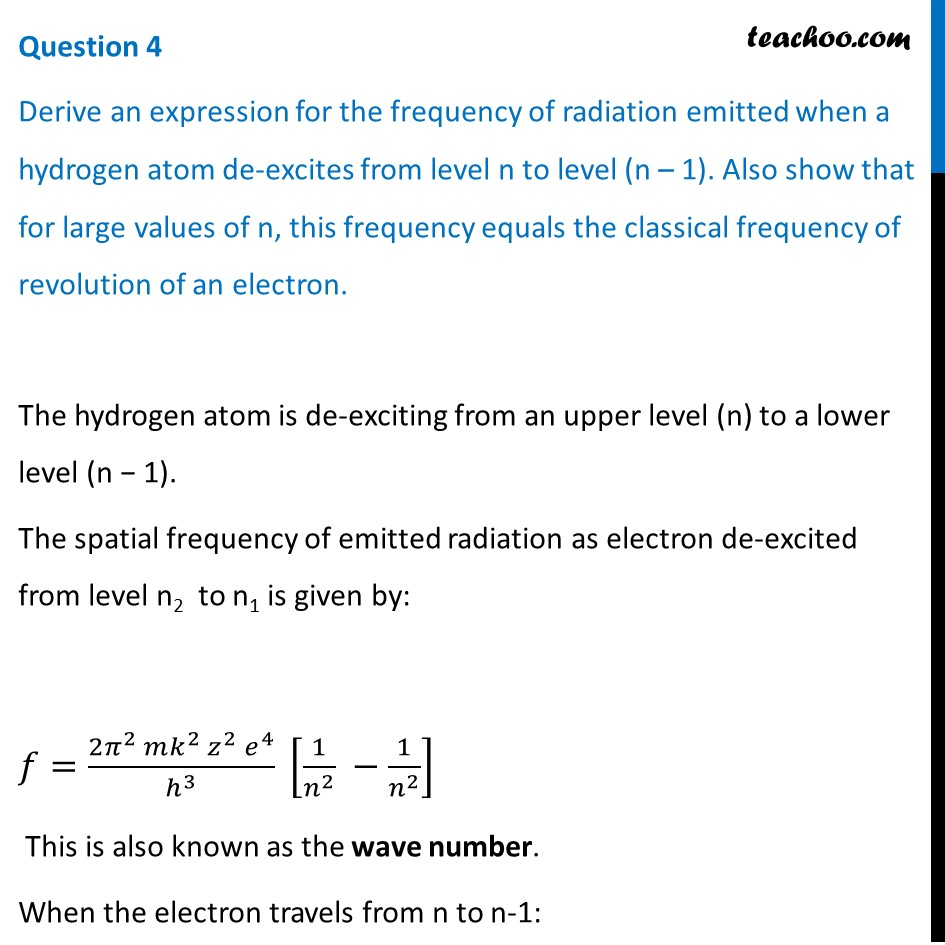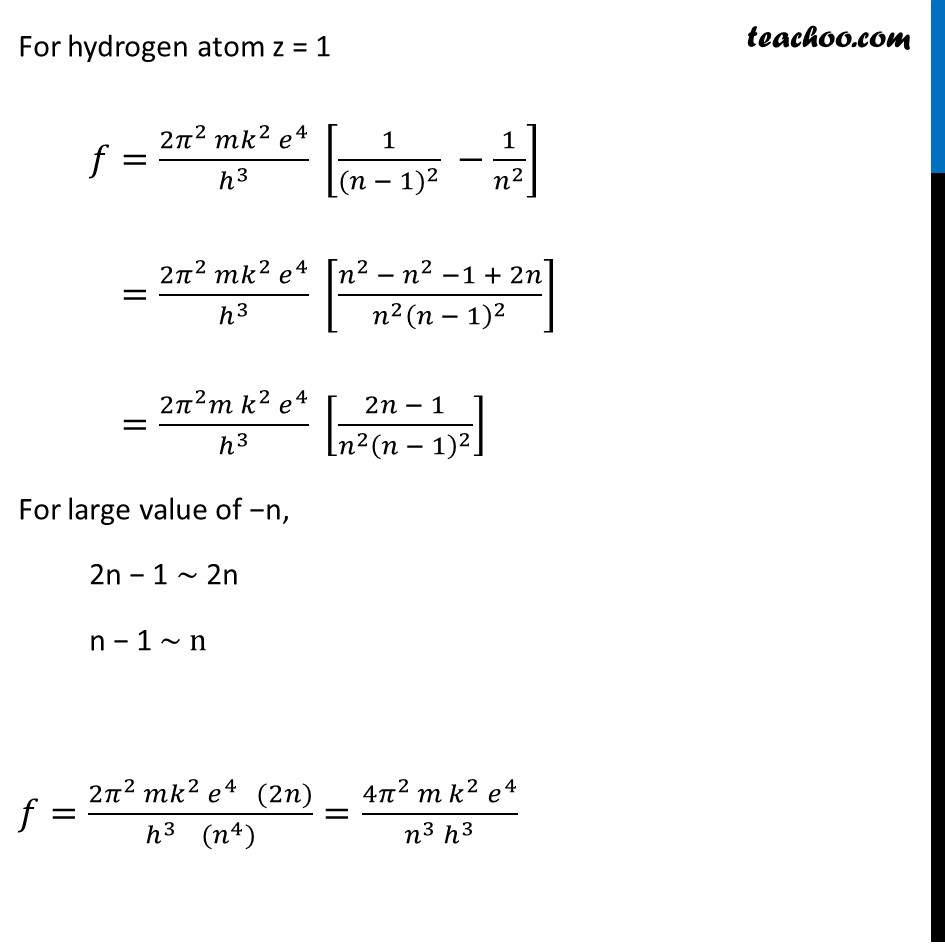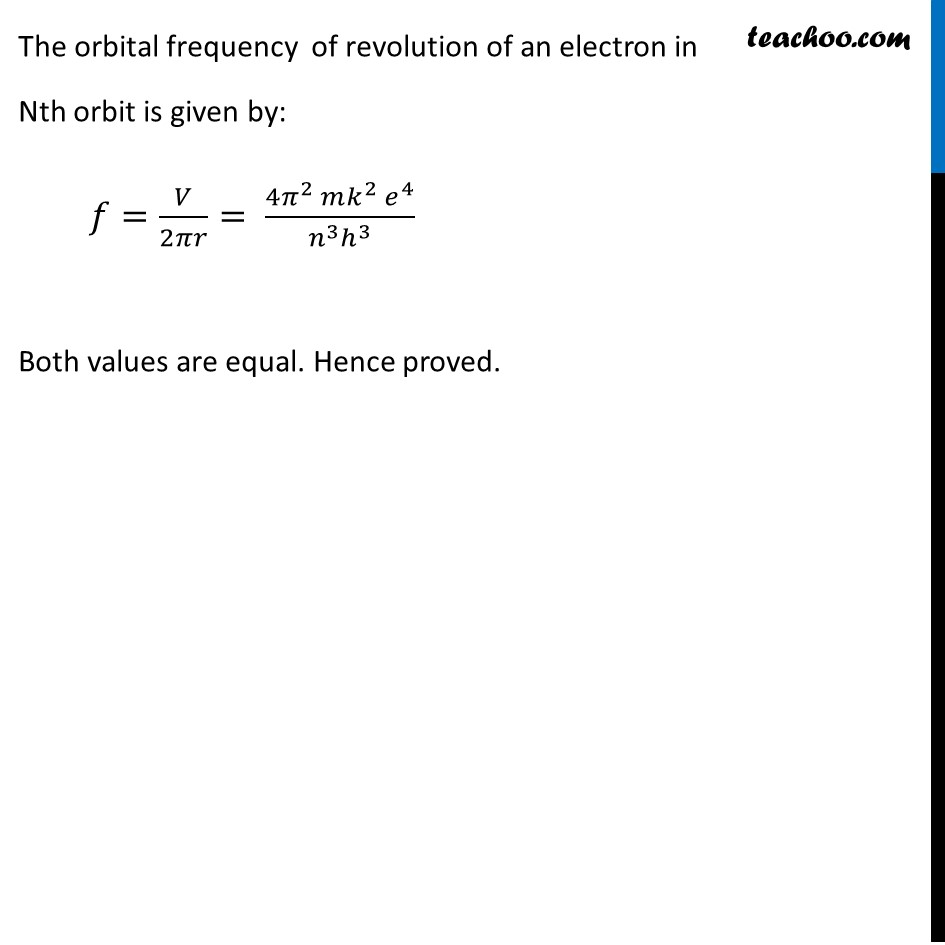CBSE Class 12 Sample Paper for 2022 Boards [Term 2] - Physics

Physics Class 12
Solutions to CBSE Sample Paper - Physics Class 12

## Derive an expression for the frequency of radiation emitted when a hydrogen atom de-excites from level n to level (n – 1). Also show that for large values of n, this frequency equals the classical frequency of revolution of an electron.Learn in your speed, with individual attention - Teachoo Maths 1-on-1 Class

### Transcript

Question 4 Derive an expression for the frequency of radiation emitted when a hydrogen atom de-excites from level n to level (n – 1). Also show that for large values of n, this frequency equals the classical frequency of revolution of an electron. The hydrogen atom is de-exciting from an upper level (n) to a lower level (n − 1). The spatial frequency of emitted radiation as electron de-excited from level n2 to n1 is given by: 𝑓=(2𝜋^2 𝑚𝑘^2 𝑧^2 𝑒^4)/ℎ^3 [1/𝑛^2 −1/𝑛^2 ] This is also known as the wave number. When the electron travels from n to n-1: For hydrogen atom z = 1 𝑓=(2𝜋^2 𝑚𝑘^2 𝑒^4)/ℎ^3 [1/〖(𝑛 − 1)〗^2 −1/𝑛^2 ] =(2𝜋^2 𝑚𝑘^2 𝑒^4)/ℎ^3 [(𝑛^2 − 𝑛^2 −1 + 2𝑛)/(𝑛^2 (𝑛 − 1)^2 )] =(2𝜋^2 𝑚 𝑘^2 𝑒^4)/ℎ^3 [(2𝑛 − 1)/(𝑛^2 (𝑛 − 1)^2 )] For large value of −n, 2n − 1 ~ 2n n − 1 ~ n 𝑓=(2𝜋^2 𝑚𝑘^2 𝑒^4 (2𝑛))/(ℎ^3 (𝑛^4))=(4𝜋^2 𝑚 𝑘^2 𝑒^4)/〖𝑛^3 ℎ〗^3 The orbital frequency of revolution of an electron in Nth orbit is given by: 𝑓=𝑉/2𝜋𝑟= (4𝜋^2 𝑚𝑘^2 𝑒^4)/(𝑛^3 ℎ^3 ) Both values are equal. Hence proved.